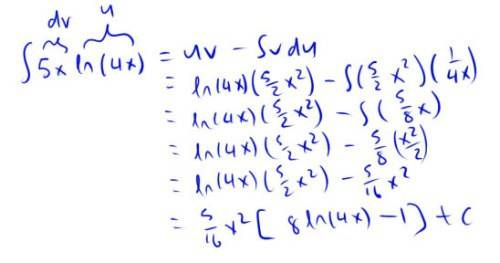# Integration by Parts 5x ln(4x)dx

## Homework Statement

Use integration by parts to evaluate the integral.
∫5x ln(4x)dx

∫udv = uv - ∫vdu

## The Attempt at a Solution

So here's my solution:But the computer is telling me I'm wrong :( We haven't learned how to integrate lnx yet, so the only choice I have is to make u = ln(4x) (even our textbook does this). Any help would be really appreciated! Thanks :)

SammyS
Staff Emeritus
Homework Helper
Gold Member

## Homework Statement

Use integration by parts to evaluate the integral.
∫5x ln(4x)dx

∫udv = uv - ∫vdu

## The Attempt at a Solution

So here's my solution:But the computer is telling me I'm wrong :( We haven't learned how to integrate lnx yet, so the only choice I have is to make u = ln(4x) (even our textbook does this). Any help would be really appreciated! Thanks :)
What is the derivative of ln(4x) ?

What is the derivative of ln(4x) ?

Oh whoops! I can't believe I didn't notice such an obvious mistake. Thanks, I got the right answer now. :)

HallsofIvy